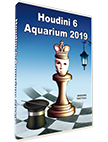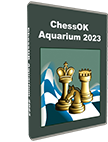﻿ Amber Rapid and Blindfold Chess Tournament 2008 - ChessOK.comHoudini Aquarium 2019 & ChessOK Aquarium 2023
ChessOK Aquarium 2023 is a new powerful analysis, database and chess publishing tool, powered by the world's strongest chess engines.

ChessOK Aquarium 2023 €39.96
Houdini 6 Aquarium 2019 €53.95

ChessOK.com » Amber Rapid and Blindfold Chess Tournament 2008
Amber Rapid and Blindfold Chess Tournament 2008 27 March 2008
 # Players 1 2 3 4 5 6 7 8 9 10 11 12 Points SB Place 1 *Aronian (2739) ** = = = = 1 = = 1 = 1 = 1 1 1 1 = = 0 = = = 1 14.5 156.3 1 2 *Kramnik (2799) = = ** 1 = 1 = = 1 = = = 0 1 = = = = = 0 = = = 12.0 133.5 2 3 *Leko (2753) = = 0 = ** 0 = = = = = = 1 = 1 = = = = 1 = = 1 12.0 128.0 3 4 *Topalov (2780) 0 = 0 = 1 = ** = = 1 0 = = 1 0 1 = = = 1 = 1 = 12.0 126.5 4 5 *Carlsen (2733) = 0 = 0 = = = = ** 0 = 1 0 = = 1 0 1 = 1 1 1 1 12.0 123.8 5 6 *Ivanchuk (2751) = 0 = = = = 0 1 1 = ** = 1 = 0 = 1 1 = = 0 = 0 11.0 120.0 6 7 *Anand (2799) = 0 = 1 = 0 = = 0 1 = 0 ** 0 1 1 = 1 0 1 = = = 11.0 117.5 7 8 *Morozevich (2765) 0 0 0 = = 0 0 1 = = = 1 1 0 ** 1 = = 1 = = 1 = 11.0 113.8 8 9 *Kariakin (2732) 0 = = = = = 0 = 0 1 = 0 0 = 0 = ** 1 = = = 1 = 9.5 101.8 9 10 *Gelfand (2737) = 1 = = = = = = 0 = 0 = 0 1 = 0 0 = ** 0 1 0 = 9.0 104.0 10 11 *Mamedyarov (2760) = = 1 = 0 = 0 = 0 0 = 1 0 = = = = = 1 0 ** 0 = 9.0 100.5 11 12 *Van Wely (2681) = 0 = = = 0 0 = 0 0 = 1 = = 0 = 0 = 1 = 1 = ** 9.0 96.0 12
List of the participants: Kramnik Vladimir (Russia, 2799), Anand Viswanathan (India, 2799), Topalov Veselin(Bulgaria, 2780), Morozevich Alexander (Russia, 2765), Mamedyarov Shakhriyaz (Azerbaijan, 2760), Leko Peter (Hungary, 2753), Ivanchuk Vassily (Ukraine, 2751), Aronian Levon (Armenia, 2739), Gelfand Boris (Israel, 2737), Carlsen Magnus (Norway, 2733), Karjakin Sergey (Ukraine, 2732), Van Wely, Loek (the Netherlands, 2681). Being held for the 17th time the well-known “Amber-tournament” is coming up this year. The tourney takes place in Nice from the 15th till the 27th of March, where 12 grand master will play the two circles system: one fast game and one blind game. Twelve grandmasters from ten different countries will take part and the total prize-fund is 216,000 Euro. Duration of the tourney: from 15th till 27th of March. Days off: 19th and 24th of March.

Option to watch the game: ONLINE Playing Zone

Study chess online on Chess King Learn! Time-proven training courses, thousands of examples and exercises.